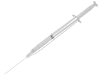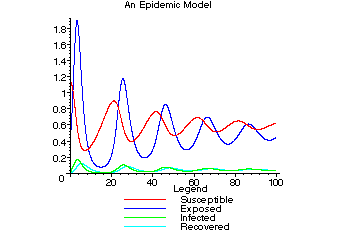Applications of Calculus to Medicine and Biology ( Math 4 ) Syllabus

This course will establish the relevance of calculus to medicine and biology. In it we will develop various mathematical tools extending the techniques of introductory calculus with a particular emphasis on the on tools needed for solving and understanding ordinary differential equations. These tools will be used to construct and explore simple and elegant models of variety of biological phenomena including the spread of an epidemic and the growth of a population. This exploration will include the examination of articles found in the current biology research literature and ample computer simulations of the models that we run into. At the heart of this course will be your own exploration of the concepts and tools introduced.

Below we see the simulation of a model describing an epidemic that arose in the paper Allometry and Simple Epidemic Models for Microparasites by G. A. De Leo and A. P. Dodson that was explored in this course.ideas